# Properties of Triangle and Height and Distance

Properties of triangle and heights and distance is a very important topic in JEE maths. We use these in finding distance between two buildings, height of a building etc. The trigonometric ratios also come into application.

Height and distance are normally used to measure the height of a building from a certain distance when the angle is measured from that point or is measured from the top of the building. In this article, we come across angle of elevation, angle of depression, line of sight and many more. This concept mostly used in determining the:

a) Distance between the two sides of the river;

b) Measurement of the height of an aeroplane from the ground flying at a constant speed;

c) Measurement of distance between the two shores of a sea;

d) Measurement of the height of a mountain.

Consider the following figure: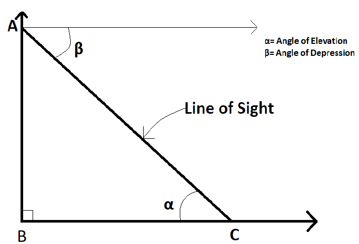The observer is at C. The tower is to be measured by the length of AB and the distance of the observer from the tower is shown by BC. The line AC represents the line of sight.

Line of Sight: The line drawn from the observer’s eye to the point observed.

Angle of Elevation: It is the angle created by the horizontal line of sight when the viewpoint is above the horizontal floor. In the figure, it is represented by the angle α.

Angle of Depression: It is the angle created by the line of sight with the horizontal point below the horizontal level. In the figure, it is represented by the angle β.

Thus we can write as: sin α = sin ∠BCA = AB/AC and tan α = tan ∠BCA = AB/BC

If we know the value of AB and BC respectively, then by using tangent angle, we can know the value of the height of the tower.

An important theorem in this topic is the m-n theorem which states: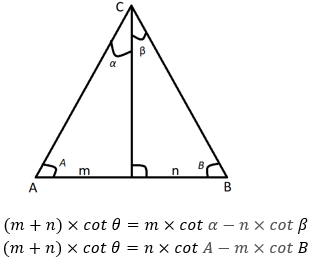## Properties of Triangle

Let us take a triangle in which we represent ∠BCA = C, ∠ABC = B, ∠BAC = A and the side opposite to CA, AB and BC be represented by b, c and a respectively. We write the perimeter as 2s and the semi-perimeter s be represented by S = (a+b+c)/2. The area of the triangle is presented by Δ. R represents the radius of the circum-circle and r represents the radius of the in-circle.

We know,

∠BCA + ∠ABC + ∠BAC = Π or C + B + A = Π

Sine Rule:

It states a/sin A = b/sin B = c/sin C = 2R

or we can write this as the sides of the triangle are proportional to the sine of the opposite angles respectively.

From this, we can also write as a = 2R × sin A, b = 2R × sin B, c = 2R × sin C

We can also write this as, a cosA + b cosB + c cosC = 4R sinA sinB sinC

This sine rule we use when two sides and one angle are given, or two angles and one side are given.

Cosine Rule:

It states

Cos A = $\frac{b^2+c^2-a^2}{2bc}$

Cos B = $\frac{a^2+c^2-b^2}{2ac}$

Cos C = $\frac{a^2+b^2-c^2}{2ab}$

This cosine rule we use when two sides and the included angle between them are given.

We can write the formulas as:

c2 = a2 + b2 – 2ab cosC

b2 = a2 + c2 – 2ac cosB

a2 = b2 + c2 – 2bc cosA

Area Formula:

Area of the triangle can be represented as:

Δ = ½ x b x c x sinA

Δ = 1/2 × c × a × sin B

Δ = 1/2 × a × b × sin C

Also, when the three sides are given, we can get the area by Heron’s formulas as:

Δ = $\sqrt{(s(s-a)(s-b)(s-c))}$

Also,

Δ = $\frac{a^2 sin\ B\ sin\ C}{2 sin\ A}=\frac{b^2 sin\ C\ sin\ A}{2 sin\ B}=\frac{c^2 sin\ A\ sin\ B}{2 sin\ C}$

Projection Rule:

This rule is used when we know two sides of a triangle and two sides and is written as

a = b × cos C + c × cos B

b = c × cos A + a × cos C

c = a × cos B + b × cos A

Circumcentre of a triangle:

It is represented by R, and it is given by:

R = abc/4Δ

R = a/(2sin A) = b/(2sin B) = c/(2sin C)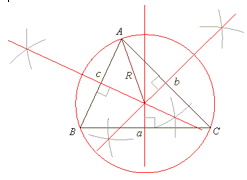Incentre of a triangle:

It is represented by r and it is given by: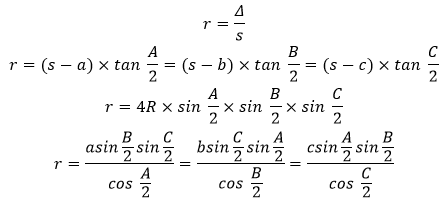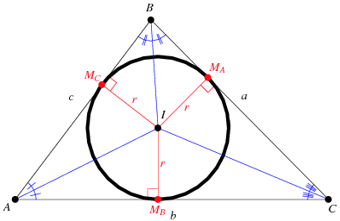Napier’s Analogy(Law of tangents):

The tangents’ law is usually described in terms of tangent formulas as follows:

(b-c)/(b+c) = (tan (B-C)/2)/(tan (B+C)/2) ;

(a-b)/(a+b)=(tan (A-B)/2)/(tan (A+B)/2) ;

(c-a)/(c+a)=(tan (C-A)/2)/(tan (C+A)/2)

Appollonius theorem:

If AD is the median of a triangle ABC then, AB2+AC2 = 2(AD 2+BD 2)

By ex-circles, we mean the circles drawn on one side which is tangent to the extensions of the other two sides and is also tangential to the side on which it is drawn.

There are three ex-circles of a triangle and their radii are represented by r1, r2, r3

Their formulas are: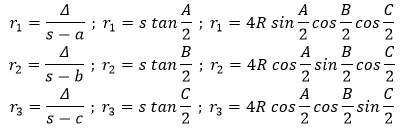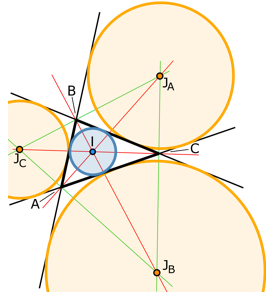Value of half-angles:

The half-angles for each of cosine, sine and tangent values are given by: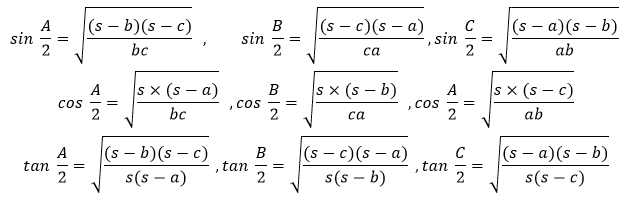### Properties and Formulas of the Isosceles Triangle

The perimeter of the isosceles triangle, P = 2x + y

The semi-perimeter of the isosceles triangle, s = P/2 = (2x+y)/2 = x + y/2

The altitude of the triangle is represented by h, and the formula is, h = √(x2 – y2/4)

The circumradius of the triangle, R = y/(2 sinα) = x/(2 sinβ)

The inradius of the triangle, r = (x-y/2) × tan α/2 = y/2 × tan β/2

Area of the triangle, A = 1/2 × y × √(x2 – y2/4) or A = 1/2 × x2 × sinα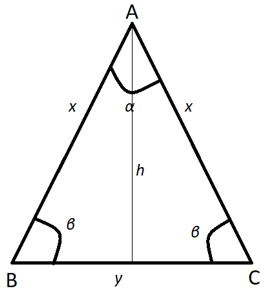### Properties and Formulas of the Equilateral Triangle

The perimeter of the isosceles triangle: P = 3c

The semi-perimeter of the isosceles triangle: s = P/2 = 3c/2

The altitude of the triangle is represented by h, and the formula is h = √(c2-c2/4) = √((4c2-c2)/4) = √(3/4) c = √3/2 c

The circumradius of the triangle, R = c/(2 × sin 60°) = c/(2×√3/2) = c/√3

The inradius of the triangle, r = (3c/2-c) × tan(60°)/2 = c/2 × tan 30° = c/2 × 1/√3 = c/(2√3)

Area of the triangle, A = 1/2 × c × √3/2 c = √3/4 c2

*Where c be the edge measure of triangle.

### Properties and Formulas of the Right-Angled Triangle

The perimeter of the isosceles triangle, P = x + y + z

The semi-perimeter of the isosceles triangle, s = P/2 = (x+y+z)/2

The circumradius of the triangle, R = z/(2×sin90°) = z/(2×1) = z/2

The inradius of the triangle, r = (s-z) × tan (90°)/2 = (s-z)×tan 45° = (s-z)×1 = (s-z)

Area of the triangle, A = 1/2 × Base × height = 1/2 xy

*Where x, y and z are sides of the right triangle.

### Expressing sines of angles in terms of sides and area

1] $sinA=\frac{2}{bc}\sqrt{s(s-a)(s-b)(s-c)}=\frac{2\Delta}{bc}$

2] $sinB=\frac{2}{ca}\sqrt{s(s-a)(s-b)(s-c)}=\frac{2\Delta}{ca}$

3] $sinC=\frac{2}{ab}\sqrt{s(s-a)(s-b)(s-c)}=\frac{2\Delta}{ab}$

### Expressing tangents and cotangents of angles in terms of sides and area

$tan\frac{A}{2}=\frac{(s-b)(s-c)}{\Delta},\ cot\frac{A}{2}=\frac{s(s-a)}{\Delta}\\ tan\frac{B}{2}=\frac{(s-c)(s-a)}{\Delta}, \ cot\frac{B}{2}=\frac{s(s-b)}{\Delta}\\ tan\frac{C}{2}=\frac{(s-a)(s-b)}{\Delta}, \ cot\frac{C}{2}=\frac{s(s-c)}{\Delta}\\$

### Important results

1] The distances between the orthocentre of the triangle and

• the vertices are 2RcosA, 2RcosB, 2RcosC and
• the sides are 2RcosB cosC, 2RcosC cosA, 2RcosA cosB

2] The circumradius of the pedal triangle = R / 2

3] Area of the pedal triangle $=2\Delta cosAcosBcosC$

4] The length of the medians of triangle ABC is given by

$AD=\frac{1}{2}\sqrt{2b^2+2c^2-a^2}\\ BE=\frac{1}{2}\sqrt{2c^2+2a^2-b^2}\\ CF=\frac{1}{2}\sqrt{2a^2+2b^2-c^2}\\$

## Solved Problems

Question 1:

From a tower, a pillar is seen. The angles of depression of the top and bottom of the pillar are x and y when seen from the top of the tower. The height of the tower is h units. Then express the height of the tower in terms of tan x and tan y.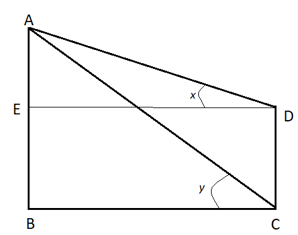Let the height of tower = h cm, and DC is the pillar.

In Δ AED,

tan x = AE/ED

And in ΔABC,

tan y = AB/BC

Now, AB=h and let us take the height of the pillar to be a.

So, BE = DC = h-a

As BEDC is a rectangle, so ED = BC

Now, (tan x)/(tan y) = AE/EB

=> (tan x)/(tan y) = a/(h-a)

=> a/h = (tan y-tan x)/(tan y)

=> a = (h(tan y-tan x))/(tan y)

So, the height of the tower is (h(tan y-tan x))/(tan y)

Question 2:

Consider a triangle ABC where AB=10 cm, BC=8 cm, CA=6 cm. A person sits at B, and his angle of elevation to the midpoint of AC is 60o. Find the distance of the person from the midpoint of AC.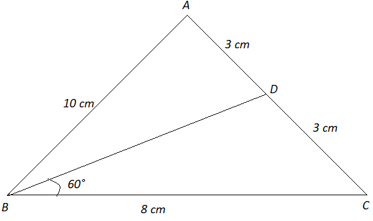To find: The distance of the person from the midpoint of AC = BD

Form ΔABC,

cos ∠BAC = $\frac{AB^2+AC^2-BC^2}{2 \times AB \times AC} = \frac{10^2+6^2-8^2}{2 \times 10 \times 6}$

Now considering ΔBDA,

cos ∠BAD = $\frac{AB^2+AD^2-BD^2}{2 \times AB \times AD}$

So,

$\frac{AB^2+AC^2-BC^2}{2 \times AB \times AC} = \frac{AB^2+AD^2-BD^2}{2 \times AB \times AD}$

=> $\frac{10^2+6^2-8^2}{2 \times 10 \times 6} = \frac{10^2+3^2-BD^2}{2 \times 10 \times 3}$

=> 72/120 = (109-BD^2)/60

=> BD2 = 109 – 73

or BD = √73

So, length of BD is √73cm. Answer!!

Example 3. A person is standing at  a distance of 40 m from a building looking at its top. The angle of elevation is of 450. Find the height of the building.

Solution: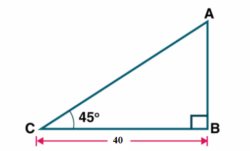Let AB be the height of the building and BC represents the distance between man and building.

Angle of elevation ∠C = 450

BC = 40 m

In triangle ABC tan 450 = AB/BC

1 = AB/40

Therefore AB = 40 m

Hence the height of the building is 40 m.